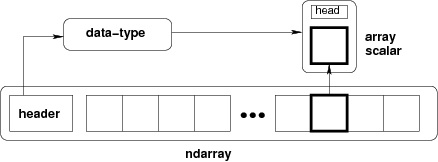MyTetra Share Делитесь знаниями!Ndarray Object
Время создания: 29.09.2017 21:28
Раздел: numPy
Запись: xintrea/mytetra_db_mcold/master/base/15067097128bhb0219i1/text.html на raw.githubusercontent.com

The most important object defined in NumPy is an N-dimensional array type called ndarray. It describes the collection of items of the same type. Items in the collection can be accessed using a zero-based index.

Every item in an ndarray takes the same size of block in the memory. Each element in ndarray is an object of data-type object (called dtype).

Any item extracted from ndarray object (by slicing) is represented by a Python object of one of array scalar types. The following diagram shows a relationship between ndarray, data type object (dtype) and array scalar type −An instance of ndarray class can be constructed by different array creation routines described later in the tutorial. The basic ndarray is created using an array function in NumPy as follows −

numpy.array

It creates an ndarray from any object exposing array interface, or from any method that returns an array.

numpy.array(object, dtype = None, copy = True, order = None, subok = False, ndmin = 0)

The above constructor takes the following parameters −

 S.No Parameter & Description 1. object Any object exposing the array interface method returns an array, or any (nested) sequence. 2. dtype Desired data type of array, optional 3. copy Optional. By default (true), the object is copied 4. order C (row major) or F (column major) or A (any) (default) 5. subok By default, returned array forced to be a base class array. If true, sub-classes passed through 6. ndimin Specifies minimum dimensions of resultant array

Take a look at the following examples to understand better.

Example 1

import numpy as np

a = np.array([1,2,3])

print a

The output is as follows −

[1, 2, 3]

Example 2

# more than one dimensions

import numpy as np

a = np.array([[1, 2], [3, 4]])

print a

The output is as follows −

[[1, 2]

[3, 4]]

Example 3

# minimum dimensions

import numpy as np

a = np.array([1, 2, 3,4,5], ndmin = 2)

print a

The output is as follows −

[[1, 2, 3, 4, 5]]

Example 4

# dtype parameter

import numpy as np

a = np.array([1, 2, 3], dtype = complex)

print a

The output is as follows −

[ 1.+0.j, 2.+0.j, 3.+0.j]

The ndarray object consists of contiguous one-dimensional segment of computer memory, combined with an indexing scheme that maps each item to a location in the memory block. The memory block holds the elements in a row-major order (C style) or a column-major order (FORTRAN or MatLab style).

Так же в этом разделе:MyTetra Share v.0.58